Updating search results...

# 5 Results

View
Selected filters:
• UT.MATH.III.F.TF.2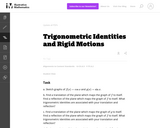Unrestricted Use
CC BY
Rating
0.0 stars

This is a task from the Illustrative Mathematics website that is one part of a complete illustration of the standard to which it is aligned. Each task has at least one solution and some commentary that addresses important asects of the task and its potential use. Here are the first few lines of the commentary for this task: Sketch graphs of $f(x) = \cos{x}$ and $g(x) = \sin{x}$. Find a translation of the plane which maps the graph of $f(x)$ to itself. Find a reflection of ...

Subject:
Mathematics
Secondary Mathematics
Material Type:
Activity/Lab
Provider:
Illustrative Mathematics
Provider Set:
Illustrative Mathematics
Author:
Illustrative Mathematics
01/06/2014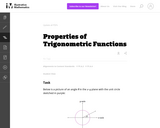Unrestricted Use
CC BY
Rating
0.0 stars

This is a task from the Illustrative Mathematics website that is one part of a complete illustration of the standard to which it is aligned. Each task has at least one solution and some commentary that addresses important asects of the task and its potential use. Here are the first few lines of the commentary for this task: Below is a picture of an angle $\theta$ in the $x$-$y$ plane with the unit circle sketched in purple: Explain why $\sin{(-\theta)} = -\sin{\theta}$ and...

Subject:
Mathematics
Secondary Mathematics
Material Type:
Activity/Lab
Provider:
Illustrative Mathematics
Provider Set:
Illustrative Mathematics
Author:
Illustrative Mathematics
01/06/2014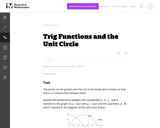Unrestricted Use
CC BY
Rating
0.0 stars

This is a task from the Illustrative Mathematics website that is one part of a complete illustration of the standard to which it is aligned. Each task has at least one solution and some commentary that addresses important asects of the task and its potential use. Here are the first few lines of the commentary for this task: The points on the graphs and the unit circle below were chosen so that there is a relationship between them. Explain the relationship between the coord...

Subject:
Mathematics
Secondary Mathematics
Material Type:
Activity/Lab
Provider:
Illustrative Mathematics
Provider Set:
Illustrative Mathematics
Author:
Illustrative Mathematics
04/01/2014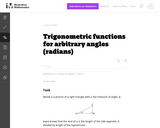Unrestricted Use
CC BY
Rating
0.0 stars

This is a task from the Illustrative Mathematics website that is one part of a complete illustration of the standard to which it is aligned. Each task has at least one solution and some commentary that addresses important asects of the task and its potential use. Here are the first few lines of the commentary for this task: Below is a picture of a right triangle with $a$ the measure of angle $A$: Joyce knows that the sine of $a$ is the length of the side opposite $A$ divid...

Subject:
Mathematics
Secondary Mathematics
Material Type:
Activity/Lab
Provider:
Illustrative Mathematics
Provider Set:
Illustrative Mathematics
Author:
Illustrative Mathematics
01/07/2014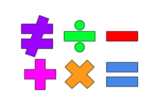Unrestricted Use
Public Domain
Rating
0.0 stars

This lesson is for a math classroom, but can be adapted to fit any grade, subject, or content.&nbsp;&nbsp;In this lesson, students will use an iPad and its features: Keynote, Pages, Garageband, Numbers, Presentation, and iMovie.&nbsp; Students will use 3 of those features/programs to create a video lesson consisting of several examples from a topic of their choice.&nbsp; This project is in place of a term final, so their chosen topic should be from their current term.Image citation: The image is one I created.&nbsp;&nbsp;

Subject:
Secondary Mathematics
Material Type:
Assessment
Author:
Tyrani Bevell